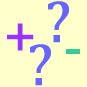#### You may also like### Prompt Cards

These two group activities use mathematical reasoning - one is numerical, one geometric.### Consecutive Numbers

An investigation involving adding and subtracting sets of consecutive numbers. Lots to find out, lots to explore.### Exploring Wild & Wonderful Number Patterns

EWWNP means Exploring Wild and Wonderful Number Patterns Created by Yourself! Investigate what happens if we create number patterns using some simple rules.

# Sums and Differences 1

## Sums and Differences 1Pick two two-digit numbers.
Find the sum of the numbers.
Find the difference between the numbers.

Can you find other numbers with the same sum and difference?

#### Why do this activity?

This activity provides a context for practising summing two two-digit numbers, and finding the difference between two two-digit numbers. It encourages working systematically as children attempt to try all possibilities to find two solutions with the same sums and differences.

#### Possible approach

It would be good to start the lesson by practising strategies for summing numbers and finding the difference between numbers.

The class could then work through a version of the challenge all together. You could model the following example and invite pairs/groups to find other pairs of numbers with the same sum:

 1st number 2nd number Sum Same? Difference Same? 24 31 55 7 15 40 55 Yes 25 No, too high

As you gather their ideas, you could present them in the table in a systematic way:

 1st number 2nd number Sum Same? Difference Same? 24 31 55 7 25 30 55 Yes 5 No, too low 26 29 55 Yes 3 No, too low

Children may notice a pattern. Will we ever find numbers with both the same sum and the same difference? Why?

Some children may move onto the extension task (below).

#### Key questions

What is the sum of the numbers? What is the difference between the numbers?
Have you tried all of the combinations? Do you need to try them all?
Can you spot a pattern?
Can you explain the pattern?

#### Possible extensions

Extension 1: Can you find pairs of numbers where the sum and difference are both odd? Can you find pairs of numbers where the sum is odd and the difference is even? Why?

Extension 2: Have a go at Sums and Differences 2. In this challenge you find the sum and difference of a pair of numbers and then find the sum and difference of the sum and difference!!

#### Possible support

A 100 square or number line/track might be useful.